## Friday, February 5, 2010

### Irodov Problem 3.15

a)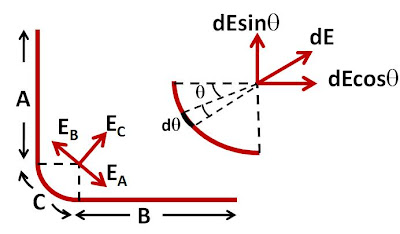We shall use the answers obtained from solution to problem 3.14 for solving this problem. Let us first divide the thread into three sections A, B and C as shown in the figure. Let the electric fields induced at the point due to each of these three sections be EA, EB and EC. The total electric field due to the entire thread is then given by EA + EB + EC. From the answer obtained in 3.14 we know the values of EA and EB as ,Clearly EA and EB are equal and opposite and will cancel each other out. So the net electric field is entire due to section C.

Now let us compute the electric field due to section C. Consider an infinitesimally small section of the thread in section C at an anglethat subtends and angleat the center. The length of this infinitesimally small section isand hence the charge contained in it is given by,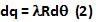The electric field generated by this infinitesimally small section (as shown in the figure) if given by,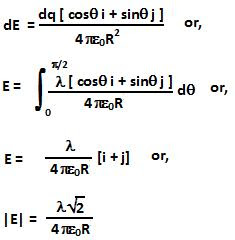b)As in case of part a) we will divide the thread into three sections A, B, and C as shown in the figure. The net electric field is given by the sum of the electric fields induced by each of the three sections. From the solution obtain in Problem 3.14 we have,The value of EC can be obtained similar to the way we obtained in part a) of this problem as,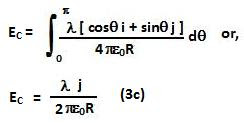From (3a), (3b) and (3c) we can see that the net sum of the three electric fields is 0.

1.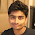1.